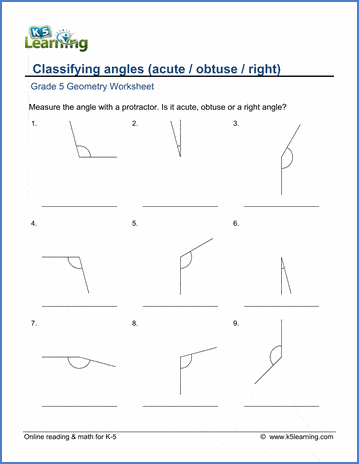The K5 Learning Blog urges parents to be pro-active in helping their children reach their full academic potential.

K5 Learning
provides free worksheets, workbooks and an online reading and math program for kindergarten to grade 5 students.# Different Types of Angles

An angle is a fraction of a circle measured in degrees. A whole circle is 360 degrees. There are four types of basic angles students in elementary school study in geometry. They are as follows.

Acute: an angle whose measure is less than 90 degrees.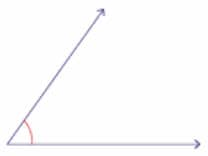Right: an angle whose measure is exactly 90 degrees.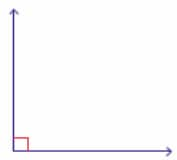Obtuse: an angle whose measure is bigger than 90 degrees but less than 180 degrees.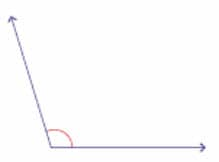Straight: an angle whose measure is 180 degrees.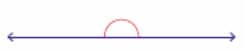## Classify angles

Before kids learn to measure angles with a protractor, they learn to classify the angles. We have created a set of grade 5 worksheets to help your child learn to classify the angles.

##Classify and measure angles

We’ve also created worksheets for classifying and measuring angles with a protractor.